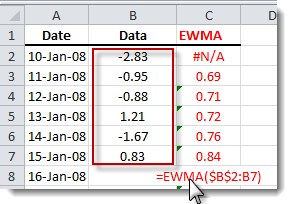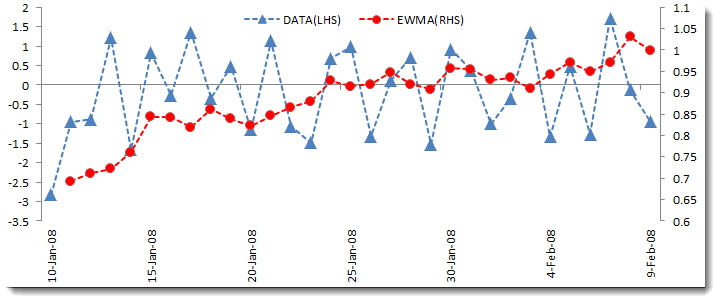# EWMA - Exponential Weighted Volatility

Calculates the estimated value of the exponentially weighted moving average (EWMA) (aka exponentially weighted volatility (EWV).

## Syntax

EWMA Excel (X, Order, Lambda, T)
X
is the univariate time series data (a one dimensional array of cells (e.g. rows or columns)).
Order
is the time order of the data series (i.e. whether the first data point corresponds to the earliest or latest date (earliest date=1 (default), latest date=0)).
Order Description
1 ascending (the first data point corresponds to the earliest date)
0 descending (the first data point corresponds to the latest date)
Lambda
is the smoothing parameter used for the exponential-weighting scheme. If missing, a default value of 0.94 is assumed.
T
is the forecast time/horizon (expressed in terms of steps beyond the end of the time series X). If missing, a default value of 0 is assumed.

## Remarks

1. The time series is homogeneous or equally spaced.
2. The time series may include missing values (e.g. #N/A) at either end.
3. If the input data set does not have a zero mean, the EWMA Excel function removes the mean from your sample data on your behalf.
4. The exponentially weighted moving average (\sigma_t) is calculated as:

$$\sigma_t^2=\lambda \sigma_{t-1}^2+(1-\lambda)x_{t-1}^2$$

Where:
• $x_t$ is the value of the time series value at time t.
• $\lambda$ is the smoothing parameter (i.e. a non-negative constant between 0 and 1).
5. The size of the EWMA Excel time series is equal to the input time series, but with the first observation (or last, if the original series is reversed) set to missing (i.e. #N/A).
6. The EWMA volatility representation does not assume a long-run average volatility, and thus, for any forecast horizon beyond one-step, the EWMA returns a constant value.

## Examples

Example 1:

See the attached spreadsheet fileThe EWMA Excel plot with the original data is shown below: